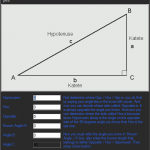# Triangle solver - with calculations v1 beta

(1 review)

## 1 Screenshot

•A triangle solver that uses the math of sine cosinus and tangens, it will also show the calculations and how the equation looks like.

If you want the picture to work put the files together in 1 folder of just put it the same place for example the desktop